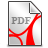# of the 1-dimensional non-linear parabolic (Stefan) model problem ...

... with not regular initial value data

We provide an ‘optimal’ finite element approximation error estimate for a one-dimensional non-linear parabolic model problem with non-regular initial value data. The solution concept is based on corresponding results of J. A. Nitsche for finite element approximation error estimates for the one-dimension Stefan problem ([NiJ], [NiJ1], [NiJ2]) leveraging on the NSE solution concept of this homepage. As the approach is not depending from the space dimension it can also be applied to the 3-D non-stationary, non-linear Navier-Stokes equations to improve the ‘not-optimal’ finite element approximation error estimates in [HeJ].

The basic idea is in line with the proposal of the "Sapce-scale turbulence" section regarding:

let ux, uxx denote the first and second derivative of the function u(x) and e the viscosity term. In (MuA) it is proposed to replace  e*uxx by -e*H[ux](x). For the latter term it holds the equality -e*H[ux](x) = e*A[uxx](x) whereby A denotes the Symm operator in the framework of L(2)-integrable periodic function on R with domain H(-1/2) (BrK1) (DeS) (MuA) (OkH).

In this case the same approach is applied to the auxiliary problem for a solution v in ([NiJ], [NiJ1], [NiJ2]) i.e. the auxiliary function v:=ux is replaced by v:=-H[ux].

For an analog NSE analysis the linearized (analog heat equation related) auxiliary problem (with corresponding 'optimal' parabolic shift theorem and related 'optimal' Ritz-Galerkin approximation estimates, [NiJ5]) needs to be replaced by the corresponding analog of the NSE theory which is about "an unusual shift theorem for Stokes flow" (see related J. A. Nitsche paper) and a corresponding "L(infinity)-analysis of the Galerkin (finite element) approximation of the solution of the Stokes equation" (J. A. Nitsche, lecture notes).

Here we areBraun K., Optimal finite element approximation estimates for non-linear parabolic problems with non regular intial value data.pdf (563.69KB)Braun K., Optimal finite element approximation estimates for non-linear parabolic problems with non regular intial value data.pdf (563.69KB)

The classical Stefan problem aims to describe the temperature distribution in a homogeneous medium undergoing a phase change, for example ice passing to water; Stefan problems are examples of free boundary problems; appropriate variable transformation leads to nonlinear parabolic initial-boundary value problems with a fixed domain.